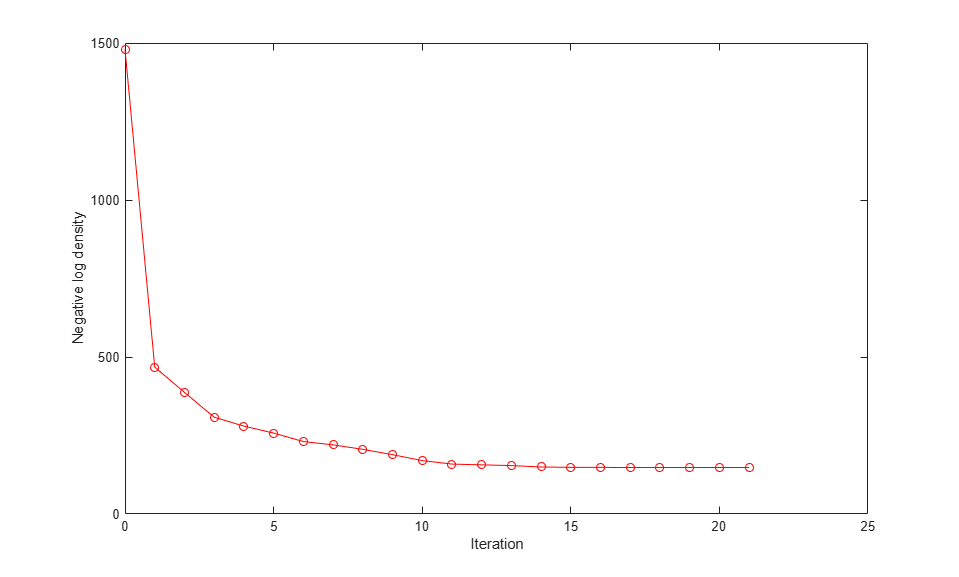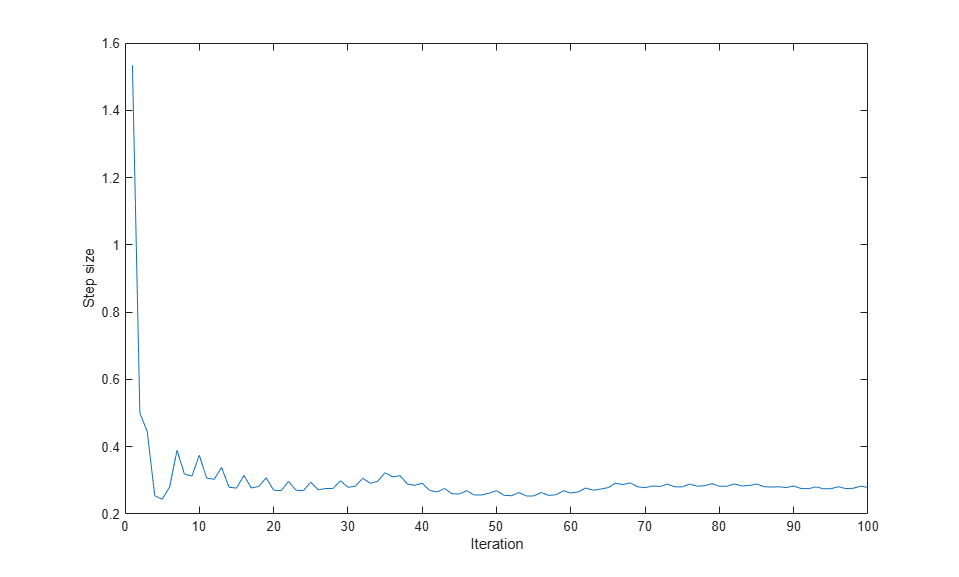# Bayesian Linear Regression Using Hamiltonian Monte Carlo

This example shows how to perform Bayesian inference on a linear regression model using a Hamiltonian Monte Carlo (HMC) sampler.

In Bayesian parameter inference, the goal is to analyze statistical models with the incorporation of prior knowledge of model parameters. The posterior distribution of the free parameterscombines the likelihood functionwith the prior distribution, using Bayes' theorem:Usually, the best way to summarize the posterior distribution is to obtain samples from that distribution using Monte Carlo methods. Using these samples, you can estimate marginal posterior distributions and derived statistics such as the posterior mean, median, and standard deviation. HMC is a gradient-based Markov Chain Monte Carlo sampler that can be more efficient than standard samplers, especially for medium-dimensional and high-dimensional problems.

### Linear Regression Model

Analyze a linear regression model with the intercept, the linear coefficients(a column vector), and the noise varianceof the data distribution as free parameters. Assume that each data point has an independent Gaussian distribution:Model the meanof the Gaussian distribution as a function of the predictorsand model parameters asIn a Bayesian analysis, you also must assign prior distributions to all free parameters. Assign independent Gaussian priors on the intercept and linear coefficients:,.

To use HMC, all sampling variables must be unconstrained, meaning that the posterior density and its gradient must be well-defined for all real parameter values. If you have a parameter that is constrained to an interval, then you must transform this parameter into an unbounded one. To conserve probability, you must multiply the prior distribution by the corresponding Jacobian factor. Also, take this factor into account when calculating the gradient of the posterior.

The noise variance is a (squared) scale parameter that can only be positive. It then can be easier and more natural to consider its logarithm as the free parameter, which is unbounded. Assign a normal prior to the logarithm of the noise variance:.

Write the logarithm of the posterior density of the free parametersasIgnore the constant term and call the sum of the last two terms. To use HMC, create a function handle that evaluatesand its gradientfor any value of. The functions used to calculateare located at the end of the script.

### Create Data Set

Define true parameter values for the intercept, the linear coefficients Beta, and the noise standard deviation. Knowing the true parameter values makes it possible to compare with the output of the HMC sampler. Only the first predictor affects the response.

NumPredictors = 2; trueIntercept = 2; trueBeta = [3;0]; trueNoiseSigma = 1; 

Use these parameter values to create a normally distributed sample data set at random values of the two predictors.

NumData = 100; rng('default') %For reproducibility X = rand(NumData,NumPredictors); mu = X*trueBeta + trueIntercept; y = normrnd(mu,trueNoiseSigma); 

### Define Posterior Probability Density

Choose the means and standard deviations of the Gaussian priors.

InterceptPriorMean = 0; InterceptPriorSigma = 10; BetaPriorMean = 0; BetaPriorSigma = 10; LogNoiseVarianceMean = 0; LogNoiseVarianceSigma = 2; 

Save a function logPosterior on the MATLAB® path that returns the logarithm of the product of the prior and likelihood, and the gradient of this logarithm. The logPosterior function is defined at the end of this example. Then, call the function with arguments to define the logpdf input argument to the hmcSampler function.

logpdf = @(Parameters)logPosterior(Parameters,X,y, ... InterceptPriorMean,InterceptPriorSigma, ... BetaPriorMean,BetaPriorSigma, ... LogNoiseVarianceMean,LogNoiseVarianceSigma); 

### Create HMC Sampler

Define the initial point to start sampling from, and then call the hmcSampler function to create the Hamiltonian sampler as a HamiltonianSampler object. Display the sampler properties.

Intercept = randn; Beta = randn(NumPredictors,1); LogNoiseVariance = randn; startpoint = [Intercept;Beta;LogNoiseVariance]; smp = hmcSampler(logpdf,startpoint,'NumSteps',50); 
smp 
smp = HamiltonianSampler with properties: StepSize: 0.1000 NumSteps: 50 MassVector: [4x1 double] JitterMethod: 'jitter-both' StepSizeTuningMethod: 'dual-averaging' MassVectorTuningMethod: 'iterative-sampling' LogPDF: [function_handle] VariableNames: {4x1 cell} StartPoint: [4x1 double] 

### Estimate MAP Point

Estimate the MAP (maximum-a-posteriori) point of the posterior density. You can start sampling from any point, but it is often more efficient to estimate the MAP point, and then use it as a starting point for tuning the sampler and drawing samples. Estimate and display the MAP point. You can show more information during optimization by setting the 'VerbosityLevel' value to 1.

[MAPpars,fitInfo] = estimateMAP(smp,'VerbosityLevel',0); MAPIntercept = MAPpars(1) MAPBeta = MAPpars(2:end-1) MAPLogNoiseVariance = MAPpars(end) 
MAPIntercept = 2.3857 MAPBeta = 2.5495 -0.4508 MAPLogNoiseVariance = -0.1007 

To check that the optimization has converged to a local optimum, plot the fitInfo.Objective field. This field contains the values of the negative log density at each iteration of the function optimization. The final values are all similar, so the optimization has converged.

plot(fitInfo.Iteration,fitInfo.Objective,'ro-'); xlabel('Iteration'); ylabel('Negative log density');### Tune Sampler

It is important to select good values for the sampler parameters to get efficient sampling. The best way to find good values is to automatically tune the MassVector, StepSize, and NumSteps parameters using the tuneSampler method. Use the method to:

1. Tune the MassVector of the sampler.

2. Tune StepSize and NumSteps for a fixed simulation length to achieve a certain acceptance ratio. The default target acceptance ratio of 0.65 is good in most cases.

Start tuning at the estimated MAP point for more efficient tuning.

[smp,tuneinfo] = tuneSampler(smp,'Start',MAPpars); 

Plot the evolution of the step size during tuning to ensure that the step size tuning has converged. Display the achieved acceptance ratio.

figure; plot(tuneinfo.StepSizeTuningInfo.StepSizeProfile); xlabel('Iteration'); ylabel('Step size'); accratio = tuneinfo.StepSizeTuningInfo.AcceptanceRatio 
accratio = 0.6700### Draw Samples

Draw samples from the posterior density, using a few independent chains. Choose different initial points for the chains, randomly distributed around the estimated MAP point. Specify the number of burn-in samples to discard from the beginning of the Markov chain and the number of samples to generate after the burn-in.

Set the 'VerbosityLevel' value to print details during sampling for the first chain.

NumChains = 4; chains = cell(NumChains,1); Burnin = 500; NumSamples = 1000; for c = 1:NumChains if (c == 1) level = 1; else level = 0; end chains{c} = drawSamples(smp,'Start',MAPpars + randn(size(MAPpars)), ... 'Burnin',Burnin,'NumSamples',NumSamples, ... 'VerbosityLevel',level,'NumPrint',300); end 
 |==================================================================================| | ITER | LOG PDF | STEP SIZE | NUM STEPS | ACC RATIO | DIVERGENT | |==================================================================================| | 300 | -1.490356e+02 | 2.617e-01 | 11 | 9.467e-01 | 0 | | 600 | -1.493478e+02 | 2.199e-02 | 3 | 9.367e-01 | 0 | | 900 | -1.509868e+02 | 2.244e-01 | 5 | 9.422e-01 | 0 | | 1200 | -1.493611e+02 | 1.166e-01 | 15 | 9.300e-01 | 0 | | 1500 | -1.488397e+02 | 2.617e-01 | 11 | 9.320e-01 | 0 | 

### Examine Convergence Diagnostics

Use the diagnostics method to compute standard MCMC diagnostics. For each sampling parameter, the method uses all the chains to compute these statistics:

• Posterior mean estimate (Mean)

• Estimate of the Monte Carlo standard error (MCSE), which is the standard deviation of the posterior mean estimate

• Estimate of the posterior standard deviation (SD)

• Estimates of the 5th and 95th quantiles of the marginal posterior distribution (Q5 and Q95)

• Effective sample size for the posterior mean estimate (ESS)

• Gelman-Rubin convergence statistic (RHat). As a rule of thumb, values of RHat less than 1.1 are interpreted as a sign that the chain has converged to the desired distribution. If RHat for any variable is larger than 1.1, then try drawing more samples using the drawSamples method.

Display the diagnostics table and the true values of the sampling parameters defined in the beginning of the example. Since the prior distribution is noninformative for this data set, the true values are between (or near) the 5th and 95th quantiles.

diags = diagnostics(smp,chains) truePars = [trueIntercept;trueBeta;log(trueNoiseSigma^2)] 
diags = 4x8 table Name Mean MCSE SD Q5 Q95 ESS RHat ______ _________ _________ _______ ________ _______ ______ ______ {'x1'} 2.3792 0.0055101 0.27821 1.9116 2.8372 2549.3 1.0009 {'x2'} 2.5533 0.0062076 0.32836 2.0223 3.0959 2798.1 1.0004 {'x3'} -0.43983 0.0065001 0.34398 -1.0192 0.12916 2800.5 1.0001 {'x4'} -0.063367 0.0028645 0.14159 -0.28853 0.1838 2443.3 1.0004 truePars = 2 3 0 0 

### Visualize Samples

Investigate issues such as convergence and mixing to determine whether the drawn samples represent a reasonable set of random realizations from the target distribution. To examine the output, plot the trace plots of the samples using the first chain.

The drawSamples method discards burn-in samples from the beginning of the Markov chain to reduce the effect of the sampling starting point. Furthermore, the trace plots look like high-frequency noise, without any visible long-range correlation between the samples. This behavior indicates that the chain is mixed well.

figure; subplot(2,2,1); plot(chains{1}(:,1)); title(sprintf('Intercept, Chain 1')); for p = 2:1+NumPredictors subplot(2,2,p); plot(chains{1}(:,p)); title(sprintf('Beta(%d), Chain 1',p-1)); end subplot(2,2,4); plot(chains{1}(:,end)); title(sprintf('LogNoiseVariance, Chain 1'));Combine the chains into one matrix and create scatter plots and histograms to visualize the 1-D and 2-D marginal posterior distributions.

concatenatedSamples = vertcat(chains{:}); figure; plotmatrix(concatenatedSamples); title('All Chains Combined');### Functions for Computing Posterior Distribution

The logPosterior function returns the logarithm of the product of a normal likelihood and a normal prior for the linear model. The input argument Parameter has the format [Intercept;Beta;LogNoiseVariance]. X and Y contain the values of the predictors and response, respectively.

The normalPrior function returns the logarithm of the multivariate normal probability density with means Mu and standard deviations Sigma, specified as scalars or columns vectors the same length as P. The second output argument is the corresponding gradient.

function [logpdf, gradlogpdf] = logPosterior(Parameters,X,Y, ... InterceptPriorMean,InterceptPriorSigma, ... BetaPriorMean,BetaPriorSigma, ... LogNoiseVarianceMean,LogNoiseVarianceSigma) % Unpack the parameter vector Intercept = Parameters(1); Beta = Parameters(2:end-1); LogNoiseVariance = Parameters(end); % Compute the log likelihood and its gradient Sigma = sqrt(exp(LogNoiseVariance)); Mu = X*Beta + Intercept; Z = (Y - Mu)/Sigma; loglik = sum(-log(Sigma) - .5*log(2*pi) - .5*Z.^2); gradIntercept1 = sum(Z/Sigma); gradBeta1 = X'*Z/Sigma; gradLogNoiseVariance1 = sum(-.5 + .5*(Z.^2)); % Compute log priors and gradients [LPIntercept, gradIntercept2] = normalPrior(Intercept,InterceptPriorMean,InterceptPriorSigma); [LPBeta, gradBeta2] = normalPrior(Beta,BetaPriorMean,BetaPriorSigma); [LPLogNoiseVar, gradLogNoiseVariance2] = normalPrior(LogNoiseVariance,LogNoiseVarianceMean,LogNoiseVarianceSigma); logprior = LPIntercept + LPBeta + LPLogNoiseVar; % Return the log posterior and its gradient logpdf = loglik + logprior; gradIntercept = gradIntercept1 + gradIntercept2; gradBeta = gradBeta1 + gradBeta2; gradLogNoiseVariance = gradLogNoiseVariance1 + gradLogNoiseVariance2; gradlogpdf = [gradIntercept;gradBeta;gradLogNoiseVariance]; end function [logpdf,gradlogpdf] = normalPrior(P,Mu,Sigma) Z = (P - Mu)./Sigma; logpdf = sum(-log(Sigma) - .5*log(2*pi) - .5*(Z.^2)); gradlogpdf = -Z./Sigma; end